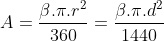# Circle sector calculator, formulas, practical use.

## Calculator for a circle sector calculations

The circle sector calculator is designed for calculations radius and diameter of a circle sector, circumference and area of a circle sector and angle and arc length of a circle sector. For successful calculation, you need to know and enter into the calculator radius or diameter and at least one of the other valuesThen press send or enter to run calculations.

## Parameters sketch:﻿
Unit system:
•

•

•          °

•

•

## Variables and constants explanation:

d – diameter
C – circumference
A – area
LA – arc length
π– 3,1415926535
β– sector angle
– unspecified values
specified values
calculated values

## Circle arc length calculation formula:## Circle sector area calculation formula:## Circle sector circumference calculation formula:## Circle sector angle calculation formula:## Calculator use:

Calculations of circle sector are used for:

• umbrella ribs
• pizza slices
• school calculations of a circle sector